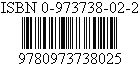A nice Website:
¤Friday the 13th¤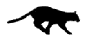HOME
 Translators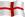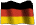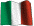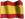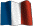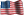Origins
 Various
 Ancients
 Modern I
 Modern II
 666: Beast
 999: Anti-Beast
 Bars Codes
 Devil's Square
 God - Trinity
 Maths 666
 References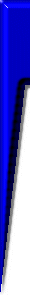χξς 666 DCLXVI וסם ﻭﺱﺥ Greek Latin Roman Hebrew Arab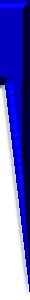Webmaster:

Ancient and Biblical Origins of Myth 666

666 in the babylonian and pagan myths

The myth of the 666 is closely related to the worship of the pagan Sun-God, practised in ancient Babylon. Advisedly Michael Scheifler underlines extracts of Roy Allan Anderson [in Unfolding the Revelation (Revised) by Roy Allan Anderson, pages 125-127, Published and copyrighted 1953, 1961,1974 by Pacific Press Publishing Association, Boise Idaho, Oshawa Ontario, Canada. Library of Congress Catalogues Card No 61-10884] which writes:
«The ancients claimed that God works by mathematics. Their religion was a conglomeration of religion, astrology, alchemy, physical and mental science, and mathematics. Ancient astrology divided the starry heavens into 36 constellations. These were represented by different amulets called "Sigilla Solis," or the sun seal. These amulets were worn by the pagan priests, and they contained all the numbers from 1 to 36. By these figures they claimed to be able to foretell future events. These amulets were usually made of gold, yellow being the sun color. While being carried, these amulets were wrapped in yellow silk, as it was thought that the bearer would thus receive the beneficial powers believed to emanate from the jewel.»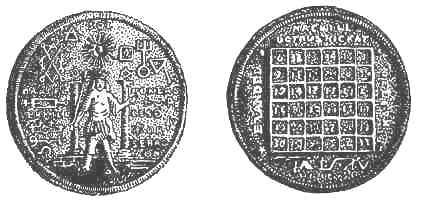«These drawings from photographs taken in 1910, show actual amulets then in the Berlin Museum. They reveal the veneration the ancients had for the sun-god. On the front side of No.1 we see the god of the sun standing on the lion. This indicated the sun's position in the constellation of Leo during the hot days of August. On the back is inscribed "Nachyel," meaning "intelligence of the sun," and in 36 squares are arranged the numerals 1 to 36 (see table 1) in such a way that adding the numbers of any column either horizontally or vertically, and also the two diagonals crossing the square, the total is the same- 111. The sum of the six columns, computed either horizontally or vertically, is 6 x 111, or 666.»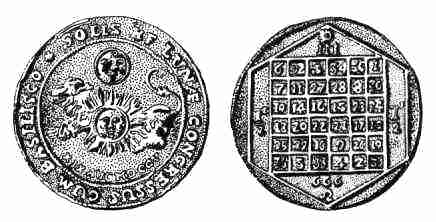«The second illustration is also a solar seal, but it honors the star Basilisco, which was the diminutive form of the Greek basileus (king), thus meaning the same as the Latin regulus. Now, Regulus is the only first-magnitude star in the constellation of Leo. The sun and the moon are again clearly seen on this amulet, and on the reverse side is the same arrangement of numerals, with the actual figure given of the total 666.»
[The lower coin shows the conjunction of the Sun, Moon and the star Regulus in the constellation of Leo the Lion that occurred on 19 August 1705.]
The magic squares below, associated with the Sun, use the thirty-six first digits and have as sum 111.
 1 32 34 3 35 6 30 8 27 28 11 7 20 24 15 16 13 23 19 17 21 22 18 14 10 26 12 9 29 25 31 4 2 33 5 36
 6 32 3 34 35 1 7 11 27 28 8 30 19 14 16 15 23 24 18 20 22 21 17 13 25 29 10 9 26 12 36 5 33 4 2 31
Tables illustrated above are also referred to in numerology as the magic Square of the Sun. There are similar magic squares for Saturn, Jupiter, Mars, Venus, Mercury and the Moon, all of which have been known for centuries.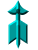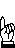666 in the Apocalypse and others biblical books

Basically and as in any REVERSE, Satan (666) is closely related to God. Just like the concept of "Light" (clear) does not mean anything in the absence of that of "Darkness" (gloomy), our white chalk on a white board actually leads to the vacuum; the ultimate solution is in a black table.

 The Apocalypse is the 66th and last book of the BIBLE. 1/66=0.0 151 515 151 515 ... ⇒ 151+515=666 The chapter 6 of the Apocalypse is well that which describes the arrival of the Antichrist. 1/6 = 1.1 666 666 666 ... ⇒ 666 «Wisdom is needed here; one who understands can calculate the number of the beast, for it is a number that stands for a person. His number is six hundred and sixty-six.» (Revelation 13/18, defines the beast; 13 symbol of dead - 18=6+6+6). Such as it clearly comes to light from the numerologic processing herewith [simple inversions, divisions and additions!], this famous verse 18 of chapter 13 of the Apocalypse codifies, at the same time and in multiple ways, the poisoned mark of the "beast" (666) and its antidote or the way of the salvation, that is "wisdom" with God (777), Jesus (8 88 888) and the anti-beast (999). Let us recall here that in greek numerology the value of Jesus [ιηςους] is: ι(10)+η(8)+ς(200)+ο(70)+υ(400)+ς(200) = 888   (8 X 111). In addition, it is important to stress that the original Greek text of the Apocalypse (book of Revelation of Jesus) comprises a total of 888 words. 13/18= 0. 7 222 222 222 ... 18/13 =1.3 846 153 846 153...13 =>1+3=4 18 =>1+8=9 13+18=3113=4+9 13=6+7 18=6+6+6=3x6=9+9 4/9= 0. 444 444 444 ... 31/13=31÷(4+9)=2.3846 153 846 ... 1/7= 0. 142 857 142 857 ... 1/9= 0. 111 111 111 ... 1/88=0.011 363 636 363 636 ... ⇒ 222+222+222=666 ⇒ 222x4= 888 ⇒ 846+153=999 ⇒ 444+444=888 ⇒ 846+153=999 ⇒ 142+857=999 ⇒ 111x6=666 111x7=777 111x8=888 111x9=999 ⇒ 363+636=999 Chapter 6 of the Gospel of John treats about the miracle of multiplication of fishes and spiritual bread, the "bread of life" (John, chap. 6/35) and consequences of the Antichrist, whose work shows through with verse 66. «As a result of this, many (of) his disciples returned to their former way of life and no longer accompanied him.» (John 6/66). 1/6=1.1 666 666 666 ... 1/66=0.0 151 515 151 515 ... 6/66 ⇒ 666 ⇒ 151+515=666 ⇒ 666 The Apocalypse is also in the center of the last chapter of the book of Isaiah, that is to say in chapter 66. «For the LORD shall judge all mankind by fire and sword; and many shall be slain by the LORD.» (Isaiah 66/16). 1/66=0.0 151 515 151 515 ... 16/66 = 0.242 424 242 424 ... 66 ⇒ 6+6=12 16 ⇒ 1+6=7 12/7=1.714 285 714 285 ... 7/12=0.58 333 333 333 ... 1/12=0.08 333 333 333 ... 1/7=0.142 857 142 857 ... ⇒ 151+515=666 ⇒ 242+424=666 ⇒ 714+285=999 333x2=666 333x3=999 333x2=666 333x3=999 ⇒ 857+142=999 The word "beast" is mentioned 37 times in the Apocalypse, in 29 verses. The numerologic processing of these two numbers reveals not only the anti-beast (999) but especially the eye of god (0/0), quite vigilant in triple 9: number 90909. The Divine Word, contained in the 66 books of the Bible, would be the way of the salvation. 37 =>3+7=10 29 =>2+9=11 37+29=66 21=10+11 10/11=0.90909 09090 90 ... 29/37=0.783 783 783 ... 15/66=0.2 272 727 272 727 ... 21/66=0.3 181 818 181 818 ... 66/21=3. 142 857 142 857 ... ⇒ 90909 ⇒ 90+909=999 ⇒ 783x2=1566 ⇒ 272+727=999 ⇒ 181+818=999 ⇒ 142+857=999 The concept of third (1÷3) has a great importance in the Apocalypse where this word appears 15 times (total of 39 occurrences in the Bible). In the book of Revelation, one finds the word "third" in Apoc. 8/7 (2 times), Apoc. 8/8 (1 time), Apoc. 8/9 (2 times), Apoc. 8/10 (1 time), Apoc. 8/11 (1 time), Apoc. 8/12 (5 times), Apoc. 9/15 (1 time), Apoc. 9/18 (1 time) and Apoc. 12/4 (1 time). The taking into account of the crowned Word (of the 39 occurrences of the third in the Bible; see link above) generates each time the anti-animal (999). 1/3=0.333 333 333 ... 2/3=0.666 666 666 ... 15/39 =0.3 846 153 846 153... 15 =>1+5=6 39 =>3+9=12 15+39=54 18=6+12 18/54=2.3 846 153 846 153 ... ⇒ 333x2=666 ⇒ 333x3=999 ⇒ 666 ⇒ 846+153=999 ⇒ 846+153=999 The BIBLE comprises 66 books, remarkable number selected for well marking the sign of the beast. 1/66=0.0 151 515 151 515 ... ⇒ 151+515=666
(BIBLE)"Wisdom": 999 integrated antidote of the 666

 "... its number is six  hundred  sixty-six " (Apocalypse 13/18) 6    ⇒ 1/6 = 0.166 666 666 666 ... 100 ⇒ 1/100 = 0.01 66   ⇒ 1/66 = 0.0 151 515 151 515 ...(⇒ 151 + 515 = 666) Generic TOTAL = 0.19 181 818 181 818 ... 181 + 818 = 999    "... here is wisdom ..." (Apocalypse 13/18) NOTE:  1/666 = 0.00 150 150 150 ...    1/999 = 0.00 100 100 100 ... 19 ⇒ 1 + 9 = 10 ⇒ 1 + 0 = 1 ⇒ Only ONE way for salvation = Jesus! ...
(BIBLE)The 7 Blisses: Way for Salvation

 1st « [...] Blessed is the one who reads aloud and blessed are those who listen to this prophetic message and heed what is written in it, for the appointed time is near.» (Apocalypse 1/3) 1/3 = 0.333 333 333 ... ⇒ 333x3=999 2nd « [...] I heard a voice from heaven say, "Write this: Blessed are the dead who die in the Lord from now on." "Yes," said the Spirit, "let them find rest from their labors, for their works accompany them." ». (Apocalypse 14/13) 14/13=1.0 769 230 769 230 ... 14 =>1+4=5 13 =>1+3=4 14+13=279 = 5+4 9/27=(5+4)÷27= 0.333 333 333 ... ⇒ 769+230=999 ⇒ 333x3=999 3rd « [...] "Behold, I am coming like a thief." Blessed is the one who watches and keeps his clothes ready, so that he may not go naked and people see him exposed !.» (Apocalypse 16/15). In this case of exception, it is the "shame" (of going naked) which generates the 666. However, the anti-beast (999) gives safety and salvation to "one who watches" regularly. 16/15=1.0 666 666 666 ... 16 =>1+6=7 15 =>1+5=6 16+15=3113 = 7+6 7/6= 1.1 666 666 666 ... 31/13=31÷(7+6)= 2.3 846 153 846 153 ... 1/7=0. 142 857 142 857 ... 1/6=0.1 666 666 666 ... ⇒ 666 ⇒ 666 ⇒ 846+153=999 ⇒ 142+857=999 ⇒ 666 4th « [...] Then the angel said to me, "Write this: Blessed are those who have been called to the wedding feast of the Lamb." And he said to me, "These words are true; they come from God."» (Apocalypse 19/9) 19/9=2.111 111 111 ... ⇒ 111x9=999 5th « [...] Blessed and holy is the one who shares in the first resurrection. The second death has no power over these; they will be priests of God and of Christ, and they will reign with him for (the) thousand years.» (Apocalypse 20/6) 20/6=10÷3= 3.333 333 333 ... ⇒ 333x3=999 6th « [...] "Behold, I am coming soon." Blessed is the one who keeps the prophetic message of this book.» (Apocalypse 22/7) 22/7=3.142 857 142 857 ... 7/22=0.3 181 818 181 818 ... ⇒ 142+857=999 ⇒ 181+818=999 7th « [...] Blessed are they who wash their robes so as to have the right to the tree of life and enter the city 9 through its gates.» (Apocalypse 22/14). Multiple "gates" for salvation (777, 888, 999) carry out in the city, but the "tree of life" is single (1). 22/14=11/7=1.5 714 285 714 22 =>2+2=4 14 =>1+4=5 22+14=369 = 4+5  36 =>3 + 6=9 4/36=0.111 111 111 ... 5/36=0.13 888 888 888 ... (4+5) ÷ (3 + 6) = 9 ÷ 9 = 1 ⇒ 714+285=999 ⇒ 111x7=777111x8=888 111x9=999 ⇒ 888
(BIBLE)

Isaiah 66 will find its principal topic in the Apocalypse, because this book is the 66th and last book of the Bible.

 1/66 = 0. 0 151 515 151 515 151 515 ... ⇒ 151 + 515 = 666

The sixth chapter of the apocalypse describes to us the arrival of the Antichrist whereas the chapter six (6) of the Gospel of John describes to us the result of the work of the Antichrist's spirit in the world ... and that in verse 66. Therefore: John 6:66 is about the Beast.666: Bivalent Myth